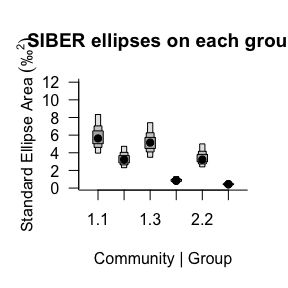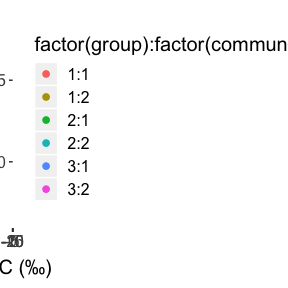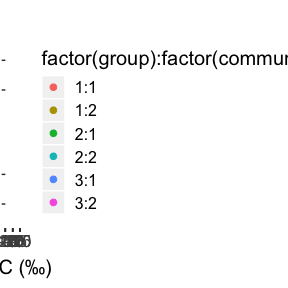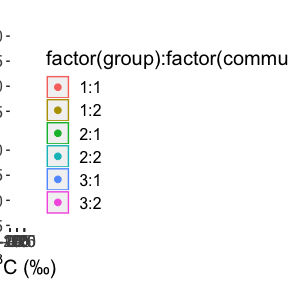Plot Some Posterior Ellipses

2019-02-22

In this example we try to create some plots of the multiple samples of the posterior ellipses using ggplot2.

library(SIBER)
library(ggplot2)
library(dplyr)
library(ellipse)
##
## Attaching package: 'ellipse'
## The following object is masked from 'package:graphics':
##
##     pairs

Fit a basic SIBER model to the example data bundled with the package.

# load in the included demonstration dataset
data("demo.siber.data")
#
# create the siber object
siber.example <- createSiberObject(demo.siber.data)

# Calculate summary statistics for each group: TA, SEA and SEAc
group.ML <- groupMetricsML(siber.example)

# options for running jags
parms <- list()
parms$n.iter <- 2 * 10^4 # number of iterations to run the model for parms$n.burnin <- 1 * 10^3 # discard the first set of values
parms$n.thin <- 10 # thin the posterior by this many parms$n.chains <- 2        # run this many chains

# define the priors
priors <- list()
priors$R <- 1 * diag(2) priors$k <- 2
priors$tau.mu <- 1.0E-3 # fit the ellipses which uses an Inverse Wishart prior # on the covariance matrix Sigma, and a vague normal prior on the # means. Fitting is via the JAGS method. ellipses.posterior <- siberMVN(siber.example, parms, priors) ## Compiling model graph ## Resolving undeclared variables ## Allocating nodes ## Graph information: ## Observed stochastic nodes: 30 ## Unobserved stochastic nodes: 3 ## Total graph size: 45 ## ## Initializing model ## ## Compiling model graph ## Resolving undeclared variables ## Allocating nodes ## Graph information: ## Observed stochastic nodes: 30 ## Unobserved stochastic nodes: 3 ## Total graph size: 45 ## ## Initializing model ## ## Compiling model graph ## Resolving undeclared variables ## Allocating nodes ## Graph information: ## Observed stochastic nodes: 30 ## Unobserved stochastic nodes: 3 ## Total graph size: 45 ## ## Initializing model ## ## Compiling model graph ## Resolving undeclared variables ## Allocating nodes ## Graph information: ## Observed stochastic nodes: 30 ## Unobserved stochastic nodes: 3 ## Total graph size: 45 ## ## Initializing model ## ## Compiling model graph ## Resolving undeclared variables ## Allocating nodes ## Graph information: ## Observed stochastic nodes: 30 ## Unobserved stochastic nodes: 3 ## Total graph size: 45 ## ## Initializing model ## ## Compiling model graph ## Resolving undeclared variables ## Allocating nodes ## Graph information: ## Observed stochastic nodes: 30 ## Unobserved stochastic nodes: 3 ## Total graph size: 45 ## ## Initializing model # The posterior estimates of the ellipses for each group can be used to # calculate the SEA.B for each group. SEA.B <- siberEllipses(ellipses.posterior) siberDensityPlot(SEA.B, xticklabels = colnames(group.ML), xlab = c("Community | Group"), ylab = expression("Standard Ellipse Area " ('\u2030' ^2) ), bty = "L", las = 1, main = "SIBER ellipses on each group" )Now we want to create some plots of some sample ellipses from these distributions. We need to create a data.frame object of all the ellipses for each group. In this example we simply take the first 10 posterior draws assuming them to be independent of one another, but you could take a random sample if you prefer. # how many of the posterior draws do you want? n.posts <- 10 # decide how big an ellipse you want to draw p.ell <- 0.95 # for a standard ellipse use # p.ell <- pchisq(1,2) # a list to store the results all_ellipses <- list() # loop over groups for (i in 1:length(ellipses.posterior)){ # a dummy variable to build in the loop ell <- NULL post.id <- NULL for ( j in 1:n.posts){ # covariance matrix Sigma <- matrix(ellipses.posterior[[i]][j,1:4], 2, 2) # mean mu <- ellipses.posterior[[i]][j,5:6] # ellipse points out <- ellipse::ellipse(Sigma, centre = mu , level = p.ell) ell <- rbind(ell, out) post.id <- c(post.id, rep(j, nrow(out))) } ell <- as.data.frame(ell) ell$rep <- post.id
all_ellipses[[i]] <- ell
}

ellipse_df <- bind_rows(all_ellipses, .id = "id")

# now we need the group and community names

# extract them from the ellipses.posterior list
group_comm_names <- names(ellipses.posterior)[as.numeric(ellipse_df$id)] # split them and conver to a matrix, NB byrow = T split_group_comm <- matrix(unlist(strsplit(group_comm_names, "[.]")), nrow(ellipse_df), 2, byrow = TRUE) ellipse_df$community <- split_group_comm[,1]
ellipse_df\$group     <- split_group_comm[,2]

ellipse_df <- dplyr::rename(ellipse_df, iso1 = x, iso2 = y)

Now to create the plots. First plot all the raw data as we want.

first.plot <- ggplot(data = demo.siber.data, aes(iso1, iso2)) +
geom_point(aes(color = factor(group):factor(community)), size = 2)+
ylab(expression(paste(delta^{15}, "N (\u2030)")))+
xlab(expression(paste(delta^{13}, "C (\u2030)"))) +
theme(text = element_text(size=15))
print(first.plot)Now we can try to add the posterior ellipses on top and facet by group

second.plot <- first.plot + facet_wrap(~factor(group):factor(community))
print(second.plot)# rename columns of ellipse_df to match the aesthetics

third.plot <- second.plot +
geom_polygon(data = ellipse_df,
mapping = aes(iso1, iso2,
group = rep,
color = factor(group):factor(community),
fill = NULL),
fill = NA,
alpha = 0.2)
print(third.plot)